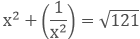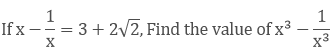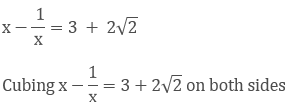×#### Thank you for registering.

One of our academic counsellors will contact you within 1 working day.

Click to Chat

1800-1023-196

+91-120-4616500

CART 0

• 0

MY CART (5)

Use Coupon: CART20 and get 20% off on all online Study Material

ITEM
DETAILS
MRP
DISCOUNT
FINAL PRICE
Total Price: Rs.

There are no items in this cart.
Continue Shopping• Complete JEE Main/Advanced Course and Test Series
• OFFERED PRICE: Rs. 15,900
• View Details

```Chapter 4: Algebraic Identities Exercise – 4.3

Question: 1

Find the cube of each of the following binomial expression

(a) (1/x + y/3)

(b) (3/x − 2/x2)

(c) (2x + 3/x)

(d) (4 − 1/3x)

Solution:

(a)  Given,

(1/x + y/3))3

The above equation is in the form of (a + b)3 = a3 + b3 + 3ab(a + b)

We know that, a = 1/x, b = y/3

By using (a + b)3  formula

(1/x + y/3))3

= (1/x)3 + (y/3)3 + 3(1/x)( y/3)(1/x + y/3)

= 1/x3 + y3/27 + 3 * 1/x * y/3(1/x + y/3)

= 1/x3 + y3/27 + y/x(1/x + y/3)

= 1/x3 + y3/27 + (y/x * 1/x) +(y/x * y3)

= 1/x3 + y3/27 + y/x2 + y2/3x)

Hence,

(1/x + y/3))3 = 1/x3 + y3/27 + y/x2 + y2/3x)

(b) Given,

((3/x−2/x2))3

The above equation is in the form of (a - b)3 = a3 - b3 - 3ab(a - b)

We know that, a = 3/x, b = 2/x2

By using (a - b)3  formula

((3/x − 2/x2))3  = (3x)3 -  (2/x2)3 - 3(3/x)( 2/x2)(3/x - 2/x2)

= 27/x3 - 8/x6 - 3 * 3/x * 2/x2(3/x - 2/x2)

= 27/x3 - 8/x6 - 18/x3(3/x - 2/x2)

= 27/x3 - 8/x6 - (18/x3 * 3/x) + (18/x3 * 2/x2)

= 27/x3 - 8/x6 - 54/x4 + 36/x5

Hence, ((3/x−2/x2))3 = 27/x3 - 8/x6 - 54/x4 + 36/x5

(c) Given,

(2x + 3/x)3

The above equation is in the form of (a + b)3 = a3 + b3 + 3ab(a + b)

We know that, a = 2x, b = 3/x

By using (a + b)3  formula

= 8x3 + 27/x3 + 18x/x(2/x + 3/x)

= 8x3 + 27/x3 + 18x/x(2x + 3/x)

= 8x3 + 27/x3 + (18 * 2x) + (18 * 3/x)

= 8x3 + 27/x3 + 36 × 54/x)

Hence,

The cube of (2x + 3/x)3 =  8x3 + 27/x3 + 36 × 54/x)

(d) Given,

(4 − 1/3x)3

The above equation is in the form of (a - b)3 = a3 - b3 - 3ab(a - b)

We know that, a = 4, b = 1/3x

By using (a - b)3  formula

(4 − 1/3x)3 = 43 - (1/3x)3 - 3(4)(1/3x)(4  - 1/3x)

= 64 - 1/27x3 - 12/3x(4 - 1/3x)

= 64 - 1/27x3 - 4/x(4 - 1/3x)

= 64 - 1/27x3 - (4/3x * 4) + (4/3x * 1/3x)

= 64 - 1/27x3 - 16/x + (4/3x2)

Hence,

The cube of (4 − 1/3x)3 = 64 - 1/27x3 - 16/x  + (4/3x2)

Question: 2

Simplify each of the following

(a) (x + 3)3 + (x - 3)3

(b) (x/2 + y/3)3 - (x/2 - y/3)3

(c) (x + 2/x)3 + (x − 2/x)3

(d) (2x - 5y)3 - (2x + 5y)3

Solution:

(a) (x + 3)3 + (x – 3)3

The above equation is in the form of a3 + b3 = (a + b)(a2 + b2 – ab)

We know that, a = (x + 3), b = (x – 3)

By using (a3 + b3)  formula

= (x + 3 + x – 3)[(x + 3)3 + (x – 3)3 – (x + 3)(x – 3)]

= 2x[(x2 + 32 + 2*x*3) + (x2 + 32 – 2*x*3) – (x2 – 32)]

= 2x[(x2 + 9 + 6x) + (x2 + 9 – 6x) – x2 + 9]

= 2x[(x2 + 9 + 6x + x2 – 9 – 6x – x2 + 9)]

= 2x(x2 + 27)

= 2x3 + 54x

Hence, the result of (x + 3)3 + (x – 3)3 is 2x3 + 54x

(b) (x/2 + y/3)3 - (x/2 – y/3)3

The above equation is in the form of a3 - b3 = (a - b)(a2 + b2 + ab)

We know that, a = (x/2 + y/3)3, b = (x/2 - y/3)3

By using (a3 - b3) formula

= [((x/2 + y/3)3 - ((x/2 − y/3)3)][((x/2 + y/3)3)2((x/2 - y/3)3)2 - ((x/2 + y/3)3)((x/2 - y/3)3)

= (x/3 + y/3 − x/2 + y/3)[((x/2)2 + (y/3)2 + (2xy/6)) + ((x/2)2 + (y/3)2 − (2xy/6)) + ((x/2)2 − (y/3)2)]

= 2y/3[(x2/4 + y2/9 + 2xy/6) + (x2/4 + y2/9 − 2xy/6) + x2/4 − y2/9]

= 2y/3[x2/4 + y2/9 + 2xy/6 + x2/4 + y2/9 − 2xy/6 + x2/4 − y2/9]

= 2y/3[x2/4 + y2/9 + x2/4 + x2/4]

= 2y/3[3x2/4 + y2/9]

= x2y/2 + 2y3/27

Hence, the result of (x/2 + y/3)3 - (x/2 - y/3)3 = x2y/2 + 2y3/27

(c)  (x + 2/x)3 + (x − 2/x)3

The above equation is in the form of a3 + b3 = (a + b)(a2 + b2 - ab)

We know that, a = (x + 2x)3, b = (x − 2x)3

By using (a3 + b3)  formula

= (x + 2/x + x − 2/x)[(x + 2/x)2 + (x − 2/x)2 − ((x + 2/x)(x − 2/x))]

= (2x)[(x2 + 4/x2 + 4x/x) + (x2 + 4/x2 − 4x/x) − (x2 − 4/x2)

= (2x)[(x2 + 4/x2 + 4x/x + x2 + 4/x2 − 4x/x − x2 + 4/x2)

= (2x)[(x2 + 4/x2 + 4/x2 + 4/x2)

= (2x)[(x2 + 12/x2)

= 2x3 + 24/x

Hence, the result of (x + 2/x)3 + (x − 2/x)3 = (2x)[(x2 + 12/x2)

(d) (2x - 5y)3 - (2x + 5y)3

Given, (2x - 5y)3 - (2x + 5y)3

The above equation is in the form of a3 - b3 = (a - b)(a2 + b2 + ab)

We know that, a = (2x - 5y), b = (2x + 5y)

By using (a3 - b3) formula

= (2x – 5y – 2x – 5y)[(2x – 5y)2 + (2x + 5y)2 + ((2x – 5y) * (2x + 5y))]

= (-10y)[(4x2 + 25y2 – 20xy) + (4x2 + 25y2 + 20xy) + 4x2 – 25y2]

= (-10y)[ 4x2 + 25y2 – 20xy + 4x2 + 25y2 + 20xy + 4x2 – 25y2]

= (-10y)[4x2 + 4x2 + 4x2 + 25y2]

= (-10y)[12x2 + 25y2}

= -120x2y – 250y3

Hence, the result of (2x – 5y)3 – (2x + 5y)3 = -120x2y – 250y3

Question: 3

If a + b = 10 and ab = 21, Find the value of a3 + b3

Solution:

Given,

a + b = 10, ab = 21

we know that, (a + b)3 = a3 + b3 + 3ab(a + b)        ... 1

substitute a + b = 10 , ab = 21  in eq 1

⟹ (10)3 = a3 + b3 + 3(21)(10)

⟹ 1000 = a3 + b3 + 630

⟹ 1000 – 630 = a3 + b3

⟹ 370 = a3 + b3

Hence, the value of a3 + b3 = 370

Question: 4

If a - b = 4 and ab = 21, Find the value of a3 - b3

Solution:

Given,

a - b = 4, ab = 21

we know that, (a - b)3 = a3 - b3 - 3ab(a - b)        -------- 1

substitute a - b = 4 , ab = 21  in eq 1

⟹ (4)3 = a3 - b3 - 3(21)(4)

⟹  64  = a3 - b3 - 252

⟹ 64 + 252 = a3 - b3

⟹ 316 = a3 - b3

Hence, the value of a3 - b3 = 316

Question: 5

If (x + 1/x) = 5, Find the value of x3 + 1/x3

Solution:

Given, (x + 1/x) = 5

We know that, (a + b)3 = a3 + b3 + 3ab(a + b)          ... 1

Substitute (x + 1/x) = 5 in eq1

(x + 1/x)3 = x3 + 1/x3 + 3(x * 1/x)(x + 1/x)

53 = x3 + 1/x3 + 3(x * 1/x)(x + 1/x)

125 = x3 + 1/x3 + 3(x + 1/x)

125 = x3 + 1/x3 + 3(5)

125 = x3 + 1/x3 + 15

125 – 15 = x3 + 1/x3

x3 +1/x3 = 110

Hence, the result is x3 + 1/x3 = 110

Question: 6

If (x − 1/x) = 7, Find the value of x3 − 1/x3

Solution:

Given, If (x − 1/x) = 7

We know that, (a - b)3 = a3 - b3 - 3ab(a - b)  ... 1

Substitute (x − 1/x) = 7 in eq 1

(x − 1/x)3 = x3 − 1/x3 - 3(x * 1/x)(x - 1/x)

73 = x3 - 1/x3 - 3(x - 1/x)

343   = x3 -1/x3 - (3 * 7)

343 = x3 - 1/x3 - 21

343 + 21 = x3 - 1/x3

x3 - 1/x3 = 364

hence, the result is x3 - 1/x3 = 364

Question: 7

If (x − 1/x) = 5, Find the value of x3 − 1/x3

Solution:

Given, If (x − 1/x) = 5

We know that, (a - b)3 = a3 - b3 - 3ab(a - b)  ... 1

Substitute (x − 1/x) = 5 in eq 1

(x − 1/x)3 = x3 - 1/x3 - 3(x * 1/x)(x - 1/x)

53 = x3 - 1/x3 - 3(x - 1/x)

125 = x3 - 1/x3 - (3 * 5)

125 = x3 - 1/x3 - 15

125 + 15 = x3 - 1/x3

x3 - 1/x3 = 140

Hence, the result is x3 - 1/x3 = 140

Question: 8

If (x2 + 1/x2) = 51, Find the value of x3 − 1/x3

Solution:

Given, (x2 + 1/x2) = 51

We know that, (x - y)2 = x2 + y2 - 2xy  .... 1

Substitute (x2 + 1/x2) = 51 in eq 1

(x - 1/x)2 = x2 + 1/x2 - 2 * x * 1/x

(x - 1/x)2  = x2 + 1/x2 - 2

(x - 1/x)2  = 51 - 2

(x - 1/x)2  = 49

(x – 1/x) =√49

(x - 1/x)  = ±7

We need to find x3 − 1/x3

So, a3 - b3 = (a - b)(a2 + b2 + ab)

x3 − 1/x3 = (x - 1/x)(x2 + 1/x2 + (x * 1/x)

We know that,

(x -1/x)  = 7 and (x2 + 1/x2) = 51

x3 − 1/x3 = 7(51 + 1)

x3 − 1/x3 = 7(52)

x3 − 1/x3 = 364

Hence, the value of x3 − 1/x3 = 364

Question: 9

If (x2 + 1/x2) = 98, Find the value of x3 + 1/x3

Solution:

Given, (x2 + 1/x2) = 98

We know that, (x + y)2 = x2 + y2 + 2xy ... 1

Substitute (x2 + 1/x2) = 98 in eq 1

(x + 1/x)2 = x2 + 1/x2 + 2 * x * 1/x

(x + 1/x)2  = x2 + 1/x2 + 2

(x + 1/x)2  = 98 + 2

(x + 1/x)2  = 100

(x + 1/x) = √100

(x + 1/x) = ± 10

We need to find x3 + 1/x3

So, a3 + b3 = (a + b)(a2 + b2 – ab)

x3 + 1/x3 = (x + 1/x)(x2 +  1/x2 - (x * 1/x)

We know that,

(x + 1/x) = 10 and (x2 + 1/x2) = 98

x3 + 1/x3 = 10(98 - 1)

x3 + 1/x3 = 10(97)

x3 + 1/x3 = 970

Hence, the value of x3 + 1/x3 = 970

Question: 10

If 2x + 3y = 13 and xy = 6, Find the value of 8x3 + 27y3

Solution:

Given, 2x + 3y = 13, xy = 6

We know that,

(2x + 3y)3 = 132

⟹ 8x3 + 27y3 + 3(2x)(3y)(2x + 3y) = 2197

⟹ 8x3 + 27y3 + 18xy(2x + 3y) = 2197

Substitute 2x + 3y = 13, xy = 6

⟹ 8x3 + 27y3 + 18(6)(13) = 2197

⟹ 8x3 + 27y3 + 1404 = 2197

⟹ 8x3 + 27y3 = 2197 – 1404

⟹ 8x3 + 27y3 = 793

Hence, the value of 8x3 + 27y3 = 793

Question: 11

If 3x - 2y = 11 and xy = 12, Find the value of 27x3 - 8y3

Solution:

Given, 3x - 2y = 11, xy = 12

We know that (a – b)3 = a3 – b3 – 3ab(a + b)

(3x - 2y)3 = 113

⟹ 27x3 – 8y3 – (18 * 12 * 11) = 1331

⟹ 27x3 – 8y3 – 2376 = 1331

⟹ 27x3 – 8y3 = 1331 + 2376

⟹ 27x3 – 8y3 = 3707

Hence, the value of 27x3 – 8y3 = 3707

Question: 12

If x4 + (1/x4) = 119, Find the value of x3 − (1/x3)

Solution:

Given, x4 + (1/x4) = 119    .... 1

We know that (x + y)2 = x2 + y2 + 2xy

Substitute x4 + (1/x4) = 119 in eq 1

(x2 + (1/x2))2 = x4 + (1/x4) + (2*x2* 1/x2)

= x4 + (1/x4) + 2

= 119 + 2

= 121

(x2 + (1/x2))2 = 121x2 + (1/x2) = ±11

Now, find (x - 1/x)

We know that (x - y)2 = x2 + y2 - 2xy

(x - 1/x)2 = x2 + 1/x2 - (2*x*1/x

= x2 + 1/x2 - 2

= 11- 2

= 9

(x – 1/x) = √9

= ±3

We need to find x3 − (1/x3)

We know that, a3 - b3 = (a - b)(a2 + b2 - ab)

x3 − (1/x3) = (x - 1/x)(x2 + (1/x2) + x * 1/x

Here, x2 + (1/x2) = 11 and (x - 1/x) = 3

x3 − (1/x3) = 3(11 + 1)

= 3(12)

= 36

Hence, the value of x3 − (1/x3) = 36

Question: 13

Evaluate each of the following

(a) (103)3

(b) (98)3

(c) (9.9)3

(d) (10.4)3

(e) (598)3

(f) (99)3

Solution:

Given,

(a) (103)3

we know that  (a + b)3 = a3 + b3 + 3ab(a + b)

⟹ (103)3 can be written as (100 + 3)3

Here, a = 100 and b = 3

(103)3 = (100 + 3)3

= (100)3 + (3)3 + 3(100)(3)(100 + 3)

= 1000000 + 27 + (900*103)

= 1000000 + 27 + 92700

= 1092727

The value of (103)3 = 1092727

(b) (98)3

we know that  (a - b)3 = a3 - b3 - 3ab(a - b)

⟹ (98)3 can be written as (100 - 2)3

Here, a = 100 and b = 2

(98)3 = (100 - 2)3

= (100)3 - (2)3 - 3(100)(2)(100 - 2)

= 1000000 - 8 - (600*102)

= 1000000 – 8 – 58800

= 941192

The value of (98)3 = 941192

(c) (9.9)3

we know that  (a - b)3 = a3 - b3 - 3ab(a - b)

⟹ (9.9)3 can be written as (10 – 0.1)3

Here, a = 10 and b = 0.1

(9.9)3 = (10 – 0.1)3

= (10)3 - (0.1)3 - 3(10)(0.1)(10 – 0.1)

= 1000 – 0.001 - (3*9.9)

= 1000 – 0.001 – 29.7

= 1000 – 29.701

= 970.299

The value of (9.9)3 = 970.299

(d) (10.4)3

we know that  (a + b)3 = a3 + b3 + 3ab(a + b)

⟹ (10.4)3 can be written as (10 + 0.4)3

Here, a = 10 and b = 0.4

(10.4)3 = (10 + 0.4)3

= (10)3 + (0.4)3 + 3(10)(0.4)(10 + 0.4)

= 1000 + 0.064 + (12*10.4)

= 1000 + 0.064 + 124.8

= 1000 + 124.864

= 1124.864

The value of (10.4)3 = 1124.864

(e) (598)3

we know that  (a - b)3 = a3 - b3 - 3ab(a - b)

⟹ (598)3 can be written as (600 - 2)3

Here, a = 600 and b = 2

(598)3 = (600 - 2)3

= (600)3 - (2)3 - 3(600)(2)(600 - 2)

= 216000000 - 8 - (3600*598)

= 216000000 - 8 - 2152800

= 216000000 - 2152808

= 213847192

The value of (598)3 = 213847192

(f) (99)3

we know that  (a - b)3 = a3 - b3 - 3ab(a - b)

⟹ (99)3 can be written as (100 - 1)3

Here , a = 100 and b = 1

(99)3  =  (100 - 1)3

= (100)3 - (1)3 - 3(100)(1)(100 - 1)

= 1000000 - 1 - (300*99)

= 1000000 - 1 - 29700

= 1000000 - 29701

= 970299

The value of (99)3 = 970299

Question: 14

Evaluate each of the following

(a) 1113 - 893

(b) 463 + 343

(c) 1043 + 963

(d) 933 - 1073

Solution:

(a) Given,

1113 - 893

the above equation can be written as (100 + 11)3 - (100 - 11)3

we know that, (a + b)3 - (a - b)3 = 2[b3 + 3ab2]

here, a = 100 b = 11

(100 + 11)3 - (100 - 11)3 = 2[113 + 3(100)2(11)]

= 2[1331 + 330000]

= 2

= 662662

The value of 1113 - 893 = 662662

(b) 463 + 343

the above equation can be written as (40 + 6)3 + (40 - 6)3

we know that, (a + b)3 + (a - b)3 = 2[a3 + 3ab2]

here, a= 40 , b = 4

(40 + 6)3 + (40 - 6)3 = 2[403 + 3(6)2(40)]

= 2[64000 + 4320]

= 2

= 1366340

The value of 463 + 343 = 1366340

(c) 1043 + 963

the above equation can be written as (100 + 4)3 + (100 - 4)3

we know that, (a + b)3 + (a - b)3 = 2[a3 + 3ab2]

here, a= 100 b = 4

(100 + 4)3 - (100 - 4)3 = 2[1003 + 3(4)2(100)]

= 2[1000000 + 4800]

= 2

= 2009600

The value of 1043 + 963 = 2009600

(d) 933 - 1073

the above equation can be written as (100 - 7)3 - (100 + 7)3

we know that, (a - b)3 - (a + b)3 = -2[b3 + 3ba2]

here, a = 93,  b = 107

(100 - 7)3 - (100 + 7)3 = - 2[73 + 3(100)2(7)]

= - 2[343 + 210000]

= - 2

= - 420686

The value of 933 - 1073 = - 420686

Question: 15

If x + 1/x = 3, calculate x2 + 1/x2, x3 + 1/x3, x4 + 1/x4

Solution:

Given, x + 1/x = 3

We know that (x + y)2 = x2 + y2 + 2xy

(x + 1/x)2 = x2 + 1/x2 + (2∗x∗1/x)

32 = x2 + 1/x2 + 2

9 - 2 = x2 + 1/x2

x2 + 1/x2 = 7

Squaring on both sides

(x2 + 1/x2)2 = 72

x4 + 1/x4 + 2* x2 * 1/x2 = 49

x4 + 1/x4 + 2 = 49

x4  + 1/x4 = 49 - 2

x4 + 1/x4 = 47

Again, cubing on both sides

(x + 1/x)3 = 33

x3 + 1/x3 + 3x*1/x(x + 1/x) = 27

x3 + 1/x3 + (3*3) = 27

x3 + 1/x3 + 9 = 27

x3 + 1/x3 = 27 - 9

x3 + 1/x3 = 18

Hence, the values are x2 + 1/x2 = 7, x4 + 1/x4 = 47, x3 + 1/x3 = 18

Question: 16

If x4 + 1/x4 = 194, calculate x2 + 1/x2, x3 + 1/x3, x + 1/x

Solution:

Given,

x4 + 1/x4 = 194                  ... 1

add and subtract (2*x2∗1/x2) on left side in above given equation

x4 + 1/x4 + (2*x2∗1/x2) - 2(2*x2∗1/x2) = 194

x4 + 1/x4 + (2*x2∗1/x2) - 2 = 194

(x2 + 1/x2)2 - 2 = 194

(x2 + 1/x2)2 = 194 + 2

(x2 + 1/x2)2 = 196(x2 + 1/x2) = 14 ... 2

Add and subtract (2*x* 1/x) on left side in eq 2

(x2 + 1/x2) + (2*x* 1/x) - (2*x* 1/x) = 14

(x + 1/x)2 - 2 = 14

(x + 1/x)2 = 14 + 2

(x + 1/x)2 = 16

(x + 1/x) = √16

(x + 1/x) = 4 ... 3

Now, cubing eq 3 on both sides

(x + 1/x)3 = 43

We know that, (a + b)3 = a3 + b3 + 3ab(a + b)

x3 + 1/x3 + 3*x*1/x(x + 1/x) = 64

x3 + 1/x3 + (3*4) = 64

x3 + 1/x3 = 64 - 12

x3 + 1/x3 = 52

hence, the values of (x2 + 1/x2)2 = 196, (x + 1/x) = 4, x3 + 1/x3 = 52

Question: 17

Find the values of 27x3 + 8y3, if

(a) 3x + 2y = 14 and xy = 8

(b) 3x + 2y = 20 and xy = 14/9

Solution:

(a) Given, 3x + 2y = 14 and xy = 8

cubing on both sides

(3x + 2y)3 = 143

We know that, (a + b)3 = a3 + b3 + 3ab(a + b)

27x3 + 8y3 + 3(3x)(2y)(3x + 2y) = 2744

27x3 + 8y3 + 18xy(3x + 2y) = 2744

27x3 + 8y3 + 18(8)(14) = 2744

27x3 + 8y3 + 2016 = 2744

27x3 + 8y3 = 2744 - 2016

27x3 + 8y3 = 728

Hence, the value of 27x3 + 8y3 = 728

(b) Given, 3x + 2y = 20 and xy = 14/9

cubing on both sides

(3x + 2y)3 = 203

We know that, (a + b)3 = a3 + b3 + 3ab(a + b)

27x3 + 8y3 + 3(3x)(2y)(3x + 2y) = 8000

27x3 + 8y3 + 18xy(3x + 2y) = 8000

27x3 + 8y3 + 18(14/9)(20) = 8000

27x3 + 8y3 + 560 = 8000

27x3 + 8y3 = 8000 - 560

27x3 + 8y3 = 7440

Hence, the value of 27x3 + 8y3 = 7440

Question: 18

Find the value of 64x3 - 125z3, if 4x - 5z = 16 and xz = 12

Solution:

Given, 64x3 - 125z3

Here, 4x - 5z = 16 and xz = 12

Cubing 4x - 5z = 16 on both sides

(4x - 5z)3 = 163

We know that, (a - b)3 = a3 - b3 - 3ab(a - b)

(4x)3 - (5z)3 - 3(4x)(5z)(4x - 5z) = 163

64x3 - 125z3 - 60(xz)(16) = 4096

64x3 - 125z3 - 60(12)(16) = 4096

64x3 - 125z3 - 11520 = 4096

64x3 - 125z3 = 4096 + 11520

64x3 - 125z3 = 15616

The value of 64x3 - 125z3 = 15616

Question: 19Solution:

Given,We know that, (a + b)3 = a3 + b3 + 3ab(a + b)```### Course Features

• 728 Video Lectures
• Revision Notes
• Previous Year Papers
• Mind Map
• Study Planner
• NCERT Solutions
• Discussion Forum
• Test paper with Video Solution